# Mean values are not the natural object to study

Moments of the Riemann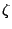-function were introduced as a tool to attack the Lindelöff Hypothesis, which asserts that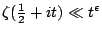for any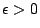. This is equivalent to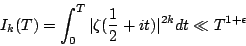for any, where the implied constant depends on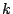.

The above bound has only been established for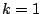and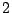, and for those values an asymptotic formula is known for the more general integral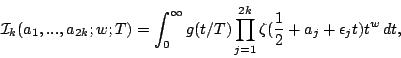where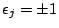and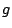is a function which descreases rapidly at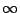.

Whenor, the function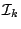can be continued to a meromorphic function. But ifis larger, thenis (conjecturally) not continuable to a meromorphic function, and in fact it has a natural boundary. Diaconu, Goldfeld, and Hoffstein have shown that the function continues to a sufficiently large region that standard conjectures for the moments of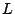-functions should be able to be recovered from the polar divisors of. However, the fact that the function under consideration is not entire suggests that it may not be the correct object to study.

Problem: Find a natural way to modifyso that it becomes an entire (meromorphic) function.

The fact thatis not a nice function for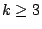is an aspect of the Estermann Phenomonon.'' Consider the Dirichlet series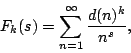where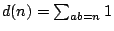is the number of divisors of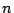. We have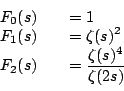But ifthen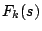can only be expressed as an infinite product of-functions, and it has a natural boundary at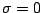.

An example which may be more closely related to the situation at hand is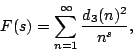where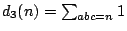. Again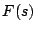has a natural boundary at, but is is possible to modify the coefficients of, only involving the terms divisible by a cube, so that the function has an analytic continuation. This is promising because a partial sum ofis the diagonal contribution to the integrand of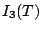.

See Titchmarsh [ MR 88c:11049] for more background information.

Back to the main index for L-functions and Random Matrix Theory.